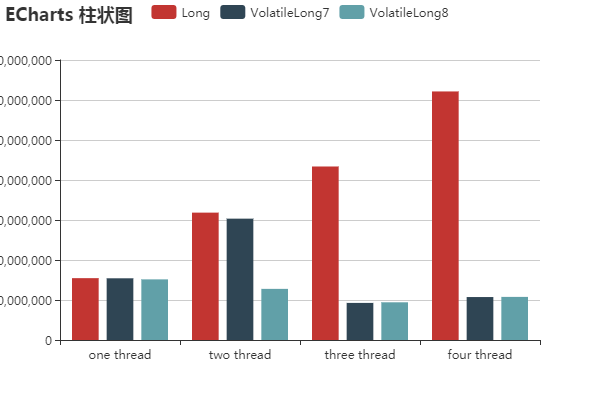伪共享（FalseSharing）图 1.

Java内存布局(Java Memory Layout)

1. doubles (8) 和 longs (8)
2. ints (4) 和 floats (4)
3. shorts (2) 和 chars (2)
4. booleans (1) 和 bytes (1)
5. references (4/8)
6. <子类字段重复上述顺序>

（译注：更多HotSpot虚拟机对象结构相关内容:http://www.infoq.com/cn/articles/jvm-hotspot

public final class FalseSharing implements Runnable
{
public final static int NUM_THREADS = 4; // change
public final static long ITERATIONS = 500L * 1000L * 1000L;
private final int arrayIndex;
private static VolatileLong[] longs = new VolatileLong[NUM_THREADS];

static
{
for (int i = 0; i < longs.length; i++)
{
longs[i] = new VolatileLong();
}
}

public FalseSharing(final int arrayIndex)
{
this.arrayIndex = arrayIndex;
}

public static void main(final String[] args) throws Exception
{
final long start = System.nanoTime();
runTest();
System.out.println("duration = " + (System.nanoTime() - start));
}

private static void runTest() throws InterruptedException
{
for (int i = 0; i < threads.length; i++)
{
}
{
t.start();
}
{
t.join();
}
}

public void run()
{
long i = ITERATIONS + 1;
while (0 != --i)
{
longs[arrayIndex].value = i;
}
}

public final static class VolatileLong
{
public volatile long value = 0L;
public long p1, p2, p3, p4, p5, p6;//, p7;// comment out
}

}